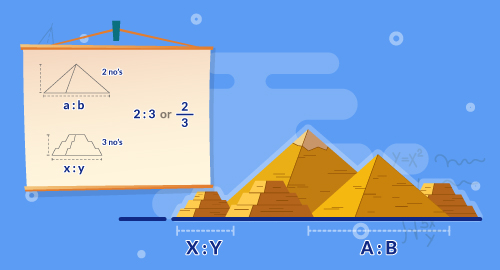#### Need Help?

Get in touch with us1-646-564-2231SAT/ACT

# Ratios, Proprortions, and Units (Fundamentals)

Maths

Understand the concept of ratio and use ratio language to describe a relationship between two quantities. Make tables of equivalent ratios relating quantities with measurement. Understand the concept of unit rate associated with a ratio a:b with b not equal to 0. Solve unit rate problems involving unit pricing and constant speed. Use ratio reasoning to convert measurement units when multiplying or dividing quantities.

##### Course Duration
•3 Weeks8 Sessions
•50 mins per session
##### Tutor## What you'll learn

• 1

Use a ratio to describe the relationship between two quantities.

• 2

Use multiplication and division to find equivalent ratios.

• 3

Compare ratios to solve them.

• 4

Solve ratio problems by using tables and graphs.

• 5

Solve problem involving rates.

• 6

Compare and use unit rates to solve problems.

• 7

Use ratio reasoning to convert customary measurements and metric measurements.

## Skills you'll learnProblem solving